Latest Banking jobs   »   Reasoning Ability Quiz For IBPS Clerk...

# Reasoning Ability Quiz For IBPS Clerk Prelims 2021- 5th August

Directions (1-5): Study the information carefully and answer the questions given below.
A person start walking in west direction from point G after walking 13m he takes a left turn and walks 7m to reach point Y. From point Y, he walks 8m in south direction to reach point H. From point H, He takes right turn and walks 6m to reach point M. Now he starts walking in east direction and walks 4m to reach at point X. From point X, He takes left turn and walks 9m to reach point L. Finally he takes a right turn and walks 15m to reach its final destination.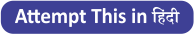Q1. What is the shortest distance between final point and G?
(a) 7m
(b) 8m
(c) 5m
(d) 4m
(e) None of these

Q2. What is the shortest distance between Point X and point Y?
(a) 2√34 m
(b) 68m
(c) 2√17m
(d)√67m
(e) None of these

Q3. In which direction point X with respect to point G?
(a) Northeast
(b) East
(c) Southwest
(d) South
(e) None of these

Q4. In which direction point Y with respect to final point?
(a) West
(b) East
(c) South
(d) Northeast
(e) None of these

Q5. If point R is 6m east of point Y then what is the shortest distance between point R and point H?
(a) 10m
(b) 8m
(c) 12m
(d) 11m
(e) None of these

Directions (6-8): Study the following information carefully and answer the questions given below:
Point A is 20m north of point B. Point F is 15m south of point E. Point G is 12m west of point H, which is mid-point of A and B. Point E is 5m east of point D. Point C is 18m west of point F. Point D is 5m north of point G.
Q6. What is the direction of point A with respect to point C?
(a) East
(b) South
(c) North-east
(d) South-west
(e) None of these

Q7. What is the shortest between E and B?
(a) 47m
(b) √31m
(c) 28m
(d) √274m
(e) None of these

Q8. What is the direction of C with respect to H?
(a) West
(b) South-west
(c) North-east
(d) North
(e) None of these

Q9. A person starts walking from point X in east direction and walks 18m to reach at point Z, from point Z he walks 4m in north direction to reach at point U. Point U is 6m east of point R, which is 8m south of point T. What is the direction of his starting point with respect to T?
(a) East
(b) North-West
(c) South-West
(d) North
(e) None of these

Directions (10-11): Study the following information carefully and answer the questions given below.
Point A is 10m West of point C. Point C is 20m South of point E. Point E is 30m West of point B. Point D is 40m South of point B. Point F is 5m South of point G. Point H is 10m West of point G. Point F is 30m West of point D.

Q10. Point H is in which direction from point A?
(a) West
(b) South west
(c) South
(d) North east
(e) South east

Q11. What is the shortest distance between Point C to Point G?
(a) 10m
(b) 20m
(c) 15m
(d) Can^’ t be determine
(e) None of these

Direction (12-15): Study the following information carefully and answer the questions given below:
Point G is in 5km north of Point E. Point B is in 8km east of Point G. Point C is in 8km west of Point H. Point B is in 14km north of Point H. Point D is in 6km west of Point F. Point A is in 5km north of Point D. Point E is in 12km east of Point A.

Q12. What is the total distance between Point A and Point B?
(a) 22km
(b) 2500m
(c) 25km
(d) 20km
(e) None of these

Q13. What is the direction of Point D with respect to Point G?
(a) South-west
(b) South-east
(c) North
(d) South
(e) North-west

Q14. What is the shortest distance between Point A and Point G?
(a) 11km
(b) 13km
(c) 14km
(d) 18km
(e) None of these

Q15. What is the direction of Point D with respect to Point C?
(a) South-east
(b) North-west
(c) South-west
(d) South
(e) North

Solutions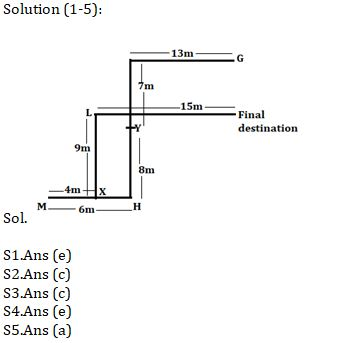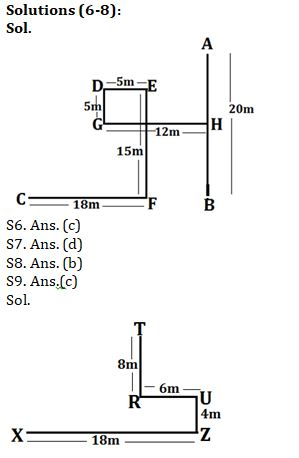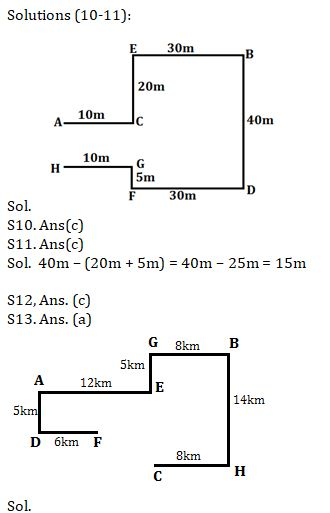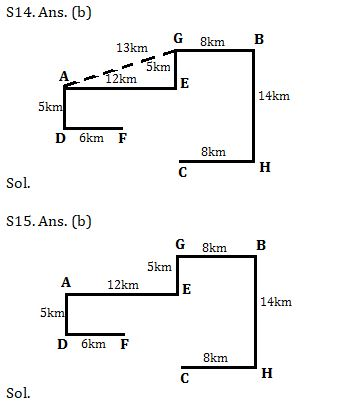#### Congratulations!Incorrect details? Fill the form again here

•Reasoning Ability Quiz For IBPS RRB PO C...
•Reasoning Ability Quiz For IBPS Clerk Pr...
•Reasoning Ability Quiz For IBPS RRB PO P...
•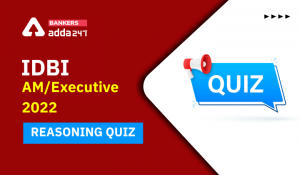Reasoning Ability Quiz For IDBI AM/Execu...
•Reasoning Ability Quiz For IBPS RRB PO P...
•Reasoning Ability Quiz For IDBI AM/Execu...